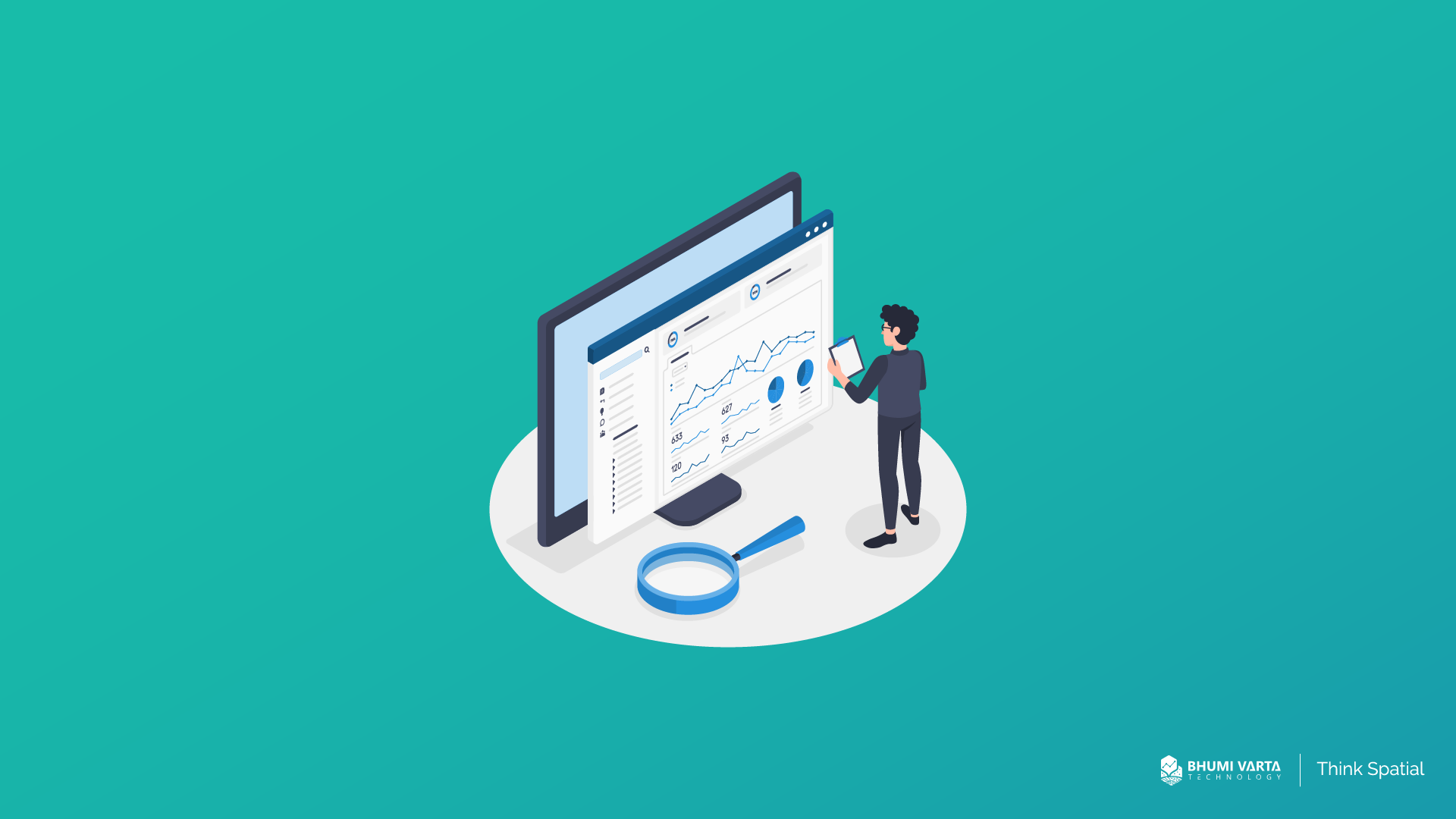Jakarta# Statistical Analysis: Understanding its Definitions, Types, and Methods

Business professionals frequently employ statistical analysis to decipher continuous trends, patterns, and other significant insights from data. This data lays the groundwork for making knowledgeable and successful decisions. In this article, you will find a comprehensive explanation of statistical analysis, including its definition, different types, and methods.

## What Is Statistical Analysis?

Discovering patterns and trends is the ultimate goal of statistical analysis, which involves gathering and interpreting data, as TechTarget stated. Interpreting research findings, conducting surveys and studies, and statistical modelling are among the various applications of statistical analysis. Additionally, it can be utilized in business intelligence to manage massive data sets.

Different outcomes can be obtained from the input data through statistical analysis. These outcomes include summarized data and significant information extracted from the input data. The result of this analysis is determined by the techniques employed.

## Types of Statistical Analysis

Two types of statistical analysis are frequently utilized: descriptive statistical analysis and inferential statistical analysis. The following are comprehensive explanations of each type.

### Descriptive Statistical Analysis

Data can be made easier to understand by collecting, organizing, analysing, and summarizing it into formats such as tables, graphs, or charts. These tasks are collectively known as descriptive statistics.

Analysts can gain a better understanding of complex data through the use of descriptive statistics. Descriptive statistical analysis can provide valuable insights, such as mode, median, mean, and variance and standard deviation measures.

### Inferential Analysis

While descriptive statistical analysis aims to summarize data, inferential analysis is employed to derive insights and draw conclusions from the analysed data. Inferential analysis draws conclusions based on a sample of data representing a more significant population. This method is often used to analyse the relationship between variables or make predictions.

It’s worth knowing that apart from the two types mentioned above, there are various types of statistical analysis.

### Predictive Analysis

Analysing past and present data to predict future events is the main purpose of this type of analysis. Techniques such as machine learning, data mining, artificial intelligence, simulation, and more are typically employed in predictive analysis.

### Prescriptive Analysis

Prescriptive analysis involves analysing data and determining the most appropriate actions based on the data’s findings. It helps businesses make decisions based on data and promotes effective decision-making in the business context.

### Exploratory Data Analysis (EDA)

Exploratory data analysis is similar to inferential analysis but focuses on exploring unknown data associations. EDA analyses potential relationships within the data and often employs statistical graphics and data visualization approaches to highlight key features.

### Causal Analysis

Causal analysis focuses on understanding the causes and effects of events. It delves into the core reasons behind events and decisions based on data analysis.

## Methods of Statistical Analysis

After comprehending their definitions and types, Let us explore the commonly used statistical analysis methods. The following are five methods that are frequently used.

### Mean

Determining the general trend of the data is the responsibility of the mean, and its calculation is simple. It includes adding up all the numbers in the data set and dividing the sum by the total number of data points. It is, however, recommended to only depend on the mean, as it may occasionally result in incorrect decision-making.

### Standard Deviation

The analysis of the deviation of individual data points from the average of the overall data set is done by standard deviation.

It establishes the spread of data in a dataset around the mean. When data points are in close proximity to the mean, the standard deviation is low, whereas it is high when they are significantly distant from it.

### Regression

The process of regression analysis involves identifying the relationships between variables and studying how dependent and independent variables are interrelated. Regression can be used to make predictions about possible future patterns and occurrences.

### Hypothesis Testing

This method tests the validity and accuracy of conclusions or arguments based on a dataset. Hypotheses are initial assumptions made at the beginning of a study and may be proven true or false based on the analysis.

### Data Sampling

Data sampling is a statistical technique used to obtain a sample from a larger population that is representative of the population as a whole. There are various methods of data sampling, including snowball sampling, random sampling, and convenience sampling.

## Identifying Sales Trends and Patterns with LOKASI Intelligence

By performing statistical analysis, organizations can determine prevalent occurrences and repeating patterns that have taken place in recent months. This data can then be leveraged by business experts to evaluate historical sales trends and employ that information to forecast future sales patterns. One of the analytical software solutions that businesses can use to gain insights like these is LOKASI Intelligence.

LOKASI Intelligence is a geospatial analytics platform that assists businesses in conducting statistical analysis to gain insights into sales trends and patterns and forecast future sales.

Additional data such as demographic information, consumer movements from telco providers, population density, socioeconomic status, points of interest, and risk data is available to enhance statistical analysis outcomes in LOKASI.

## FAQ

### What are the types of statistical analysis?

Statistical analysis can be broadly classified into six types: descriptive, inferential, predictive, prescriptive, exploratory data analysis (EDA), and causal analysis.

### What is statistical analysis?

Statistical analysis is the collection and interpretation of data to discover patterns and trends.

### What are the methods used in statistics?

Five statistical analysis techniques are frequently used, including Mean, Standard Deviation, Regression, Hypothesis Testing, and Data Sampling.